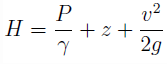Courses

# Test: Hydraulic Gradient & Total Energy Line

## 10 Questions MCQ Test Fluid Mechanics | Test: Hydraulic Gradient & Total Energy Line

Description
This mock test of Test: Hydraulic Gradient & Total Energy Line for Civil Engineering (CE) helps you for every Civil Engineering (CE) entrance exam. This contains 10 Multiple Choice Questions for Civil Engineering (CE) Test: Hydraulic Gradient & Total Energy Line (mcq) to study with solutions a complete question bank. The solved questions answers in this Test: Hydraulic Gradient & Total Energy Line quiz give you a good mix of easy questions and tough questions. Civil Engineering (CE) students definitely take this Test: Hydraulic Gradient & Total Energy Line exercise for a better result in the exam. You can find other Test: Hydraulic Gradient & Total Energy Line extra questions, long questions & short questions for Civil Engineering (CE) on EduRev as well by searching above.
QUESTION: 1

### Energy gradient line takes into consideration

Solution:

Explanation: EGL is obtained by plotting total head at various points along the axis of the pipe.where H is the total head, P / γ is the pressure head, z is the potential head and v2 / 2g is the velocity head. Hence, EGL is also called Total Energy Line (TEL).

QUESTION: 2

### Hydraulic gradient line takes into consideration

Solution:

Explanation: HGL is obtained by plotting piezometric head at various points along the axis of the pipe.
Hp = P ⁄ γ + z
where Hp is the piezometric head, P ⁄ γ is the pressure head and z is the potential head.

QUESTION: 3

### Which of the following is true?

Solution:

Explanation: EGL is obtained by plotting total head at various points along the axis of the pipe. Since the total head decreases in the direction of flow, EGL will always drop in that direction.

QUESTION: 4

Which of the following is true?

Solution:

Explanation: HGL is obtained by plotting piezometric head at various points along the axis of the pipe. Since pressure may either rise or fall in the direction of flow, HGL may or may not change in that direction.

QUESTION: 5

Which of the following is true?

Solution:

Explanation: EGL is obtained by plotting total head and HGL is obtained by plotting piezometric head at various points along the axis of the pipe.Hp = P ⁄ γ + z
where H is the total head, P ⁄ γ is the pressure head, z is the potential head, Hp is the piezometric head, and v2 / 2g is the velocity head.
H = Hp + v2 / 2g Since Hp < H, HGL can never be above EGL.

QUESTION: 6

The vertical intercept between EGL and HGL is equal to

Solution:

Explanation: EGL is obtained by plotting total head and HGL is obtained by plotting piezometric head at various points along the axis of the pipe.Hp = P ⁄ γ + z
where H is the total head, P ⁄ γ is the pressure head, z is the potential head, Hp is the piezometric head, and v2 / 2g is the velocity head.
H – Hp = v2 / 2g, the vertical intercept between EGL and HGL is equal to the kinetic head.

QUESTION: 7

The slope of HGL will be

Solution:

Explanation: The vertical intercept between EGL and HGL is equal to the kinetic head. For a pipe of uniform cross-section, there will be no change in the velocity of flow across the pipe. Since the kinetic head remian constant, the slope of HGL will be equal than that of EGL.

QUESTION: 8

For a nozzle, the vertical intercept between EGL and HGL

Solution:

Explanation: The vertical intercept between EGL and HGL is equal to the kinetic head. For a nozzle, the cross-sectional area decreases in the direction of flow leading to an increase in the velocity of flow across the pipe. Since the kinetic head increases, the vertical intercept between EGL and HGL will increase.

QUESTION: 9

For a diffuser, the vertical intercept between EGL and HGL

Solution:

Explanation: The vertical intercept between EGL and HGL is equal to the kinetic head. For a diffuser, the cross-sectional area increases in the direction of flow leading to a decrease in the velocity of flow across the pipe. Since the kinetic head decreases, the vertical intercept between EGL and HGL will decrease.

QUESTION: 10

Which of the following is true?

Solution:

Explanation: EGL is obtained by plotting total head at various points along the axis of the pipe.where H is the total head, P ⁄ γ is the pressure head, z is the potential head, , and v2 / 2g is the velocity head.
Hence, there is no relation whatsoever between the slope of EGL and that of the axis of the pipe.